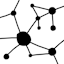#Animated floating graph nodes

## Demo (JavaScript)

 Number of nodes: Extra edges: % Network style: Mesh Balanced Hub-and-spoke Drift speed: Repulsion force:

(Note: If your browser doesn’t support SVG or JavaScript, here is a static image as an example.)

## Description

In this mini-project, art meets computer science. I was asked by a client to make an animation of graph nodes and edges floating in space. The project involved writing a program to achieve an aesthetic result. Below I will describe how the code works, starting from a rough sketch and refining the details.

We start off with a blank rectangular image, and place a number of new graph nodes onto it at random positions with random radii. We also give them random velocities so they drift around. As nodes drift past the edge of the image, they fade out and disappear. New random nodes are created within the image bounds to keep the total number of nodes stable.

Clumpiness is a problem when working with uniform random numbers. Some areas of the graph are too dense with nodes while others are empty. We want an image that is mostly uniform in the density of nodes. To achieve this, we introduce a “force field” that makes nodes repel each other. On every frame, we apply the inverse-square law to move the positions of nodes away from each other. (Real physics use the inverse-square law to change the velocities, but I prefer to change the positions because the effect is softer.)

To make the graph into a network, we need to add some edges. We take the brute-force approach of constructing a minimum spanning tree over all the nodes, based on the Euclidean distance metric. We add these MST nodes to the graph and draw them.

To make things more interesting, we skew the distance metric somewhat. The plain distance metric yields a mesh-like MST. If we divide the distance by the product of the radii of both endpoints, we get a star-like hub-and-spoke network. If we divide by the square root of the product of radii, we get something in between. Also, we add some redundant edges to the graph in addition to the MST; we select a bunch of low-weight edges that are not already in the set of MST edges.

If we implemented the algorithm up to this point in a literal way, the edges would flicker on and off as the minimum spanning tree changes. To make the animation smoother, we give each existing edge an opacity value between 0.0 and 1.0. On every frame we compute the MST and extra edges – which we call the “ideal set” – and if an existing edge is in the ideal set then its opacity increases; otherwise its opacity decreases. When an edge’s opacity decreases to zero, we remove it and allow new edges in the ideal set to be added.

Embarrassingly the code uses O(V2) algorithms in about a dozen places, so it is clearly not scalable to a large number of nodes. For the purposes of making a cute “screensaver” animation, about 100 nodes are sufficient and tractable. If more nodes are desired while keeping the same flavor of the algorithm, then it is probably necessary to implement strategies like binary space partitioning, heuristic pruning of the search space, and infrequent updates for expensive operations.